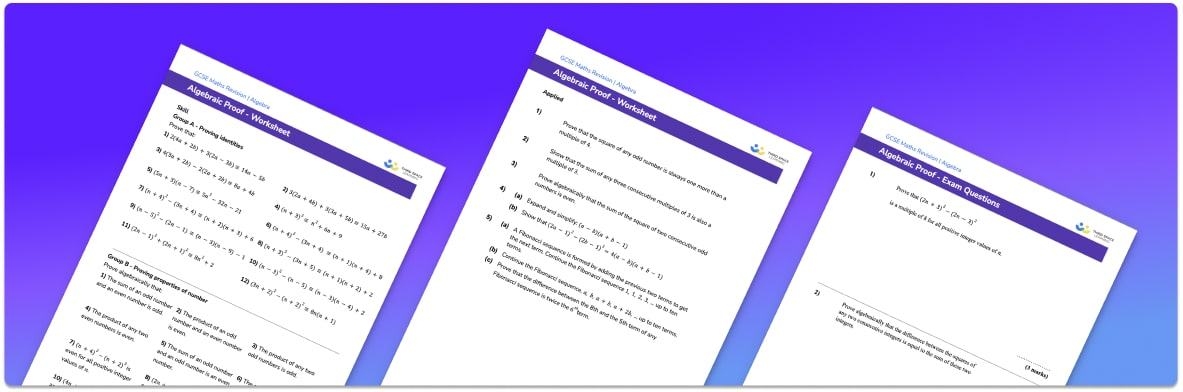# Algebraic Proof Worksheet• Section 1 of the algebraic proof  worksheet contains 20+ skills-based algebraic proof  questions, in 3 groups to support differentiation
• Section 2 contains 3 applied algebraic proof  questions with a mix of worded problems and deeper problem solving questions
• Section 3 contains 3 foundation and higher level GCSE exam style algebraic proof  questions
• Answers and a mark scheme for all algebraic proof  questions are provided
• Questions follow variation theory with plenty of opportunities for students to work independently at their own level
• All questions created by fully qualified expert secondary maths teachers
• Suitable for GCSE maths revision for AQA, OCR and Edexcel exam boards

• This field is for validation purposes and should be left unchanged.

You can unsubscribe at any time (each email we send will contain an easy way to unsubscribe). To find out more about how we use your data, see our privacy policy.

### Algebraic proof at a glance

An algebraic proof is a logical sequence of statements that proves that something is always true. For example, we could use algebra to prove that the sum of two consecutive odd numbers is always a multiple of 4 or that the sum of the squares of two consecutive integers (whole numbers) is always odd.

Algebraic proofs involve working from a starting point, and often require the expansion of brackets followed by the manipulating/simplifying/factorising of the algebraic expression until a conclusion can be made about that expression.

When working on proofs, we generally use 2n when we want to represent an even number (since 2 times any number is always even) and 2n+1 to represent an odd number (since 1 more than an even number is always odd). We could use 2n+2, 2n+4, … for further even numbers and 2n+3, 2n+5,… for further odd numbers.

Mathematical proof is studied further in A Level maths, and other methods of proof are introduced.

Looking forward, students can then progress to additional proofs in maths worksheets and other algebra worksheets, for example a sequences worksheet, simultaneous equations worksheet or straight line graphs worksheet.For more teaching and learning support on Algebra our GCSE maths lessons provide step by step support for all GCSE maths concepts.

## Do you have KS4 students who need more focused attention to succeed at GCSE?There will be students in your class who require individual attention to help them succeed in their maths GCSEs. In a class of 30, it’s not always easy to provide.

Help your students feel confident with exam-style questions and the strategies they’ll need to answer them correctly with our dedicated GCSE maths revision programme.

Lessons are selected to provide support where each student needs it most, and specially-trained GCSE maths tutors adapt the pitch and pace of each lesson. This ensures a personalised revision programme that raises grades and boosts confidence.

Find out more